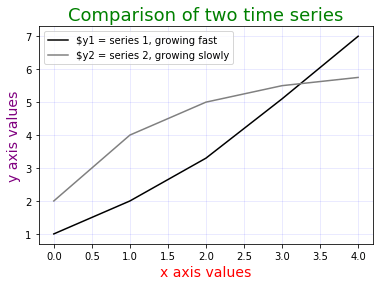# Python 中的 Matplotlib 简介```# 导入 matplotlib，matplotlib.pyplot
import matplotlib
import matplotlib.pyplot as plt
# 导入 pandas 和numpy，因为我们想可视化存储在数据框中的数据
import pandas
import numpy
# 导入数据集并显示标题
```
```# 第一步：创建表面，图形；允许例如设置大小
# 图形就像一页；一个图形可以包含多个图，即轴
plt.figure(figsize=(10,10))
```
`<图尺寸 720x720，0 轴>`
`<图尺寸 720x720，0 轴>`
```# .subplots() 返回图形和坐标轴；
# 坐标轴是你绘制的基础坐标；
# 如前所述，一个图形可以包含多个轴
plt.figure(figsize=(10,10))
plt.subplots()
```
```(<Figure size 432x288 with 1 Axes>,
<matplotlib.axes._subplots.AxesSubplot at 0x257b791bb08>)```
`<图尺寸 720x720，0 轴>`
```# step 2: 绘制点图，即散点图；
# 将绘图添加到轴对象
plt.figure(figsize=(10,10))
plt.plot( numpy .sort(data_df["output "]),marker="o",markersize=2)
```
`[<matplotlib.lines.Line2D 位于 0x257b6180108>]`
```# 第三步：添加标题和坐标轴标签；
# -- 设置图形大小
plt.figure(figsize=(20,10))
# -- 创建绘图
plt.plot( numpy .sort(data_df["output "]),
marker="o",
markersize=6,
linewidth =2,
linestyle ="--",
color="orange")
# -- set title
plt.title("汽车行业年产量数字",
fontdict={"fontname":"Times New Roman",
"fontsize" :32})
# -- 赋值 xlabel
plt.xlabel("data point no.",
fontdict={"fontname":"Comic Sans MS",
fontsize=18,
color="green")
# -- 调整xticks
plt.xticks(size=16,
color="purple")
```
```(array([-200., 0., 200., 400., 600., 800., 1000.]),
<7 个文本 xticklabel 对象的列表>)```
```# 使用 matplotlib 的一种更结构化的方式是使用引用处理程序
# -- 设置一些要绘制的数据向量
y1 = [1,2,3.3,5.1,7]
y2 = [2,4,5,5.5, 5.75]
x = range(0,len(y1))
# -- 创建一个空图片（即=图）；捕获一个处理程序
fig = plt.figure() # fig 表示这是一个“图形”
# -- 在空图片上创建一个子图，即空图形；捕获一个处理程序
ax = plt.subplot() # ax 表示这是一个“轴”；坐标轴基本上是图表
# -- 在坐标轴上创建线图，使用坐标轴处理程序参考
ax.plot(x, y1, label='\$y1 = series 1, growing fast',color="black")在轴上创建线图
# - - 为轴添加标题，
ax.set_title('Comparison of two time series',
fontsize=18,
color="green")
# -- 添加 x 和 y 轴标签，使用轴处理程序引用
ax.set_xlabel("x 轴值",
fontsize=14,
color="red")
ax.set_ylabel("y axis values",
fontsize=14,
color="purple")
# -- 添加图例，默认情况下在图框内
ax.legend(fontsize=10)
# -- 添加一个grid
ax.grid(b=True,
color="blue",
alpha=0.1)
# -- 显示本节中绘制的所有内容，直到此时
plt.show()
```
```# 现在让我们看一些额外的例子；
# 例如，我们可以使用 matplotlib 制作直方图
# -- 导入 random 以创建一些随机数
import random
# -- 使用 random 中的 randint() 来创建一些随机整数
x = []
for i in range(0,100):
x.append( random.randint(a=0,b=100))
# -- 创建一个图形
fig = plt.figure(figsize=(10,10))
# -- 添加轴到图形
ax = plt.subplot()
# --使用轴对象处理程序向轴添加直方图
ax.hist(x,
bins=20,
histt​​ype="bar",
color="pink")
# -- 使用轴对象处理程序向直方图添加标题
ax.set_title("a histogram, created with matplotlib in Python",
fontsize=22,
color="darkgreen")
# -- 添加标签到 x 和 y 轴，使用轴对象处理程序
ax.set_xlabel("observation value range",
fontsize=16,
color="darkgreen")
ax.set_ylabel("absolute frequency",
fontsize=16,
color="darkgreen")
# -- 调整 x 和 y 刻度标签，使用轴对象处理程序
# -- 也：调整 x 和 y 轴刻度本身，使用轴对象处理程序
ax.tick_params(axis="x" ，
尺寸=12，
宽度=5，
颜色=“蓝色”，
标签尺寸=20，
宽度=5，
颜色=“蓝色”）
ax.tick_params(axis="y",
size=12,
# -- 显示本节绘制的所有内容，直到此时
plt.show()
```
```# 另一个例子：在 Python 中使用 matplotlib 绘制 3D 表面图
# credit: https://stackoverflow.com/questions/3810865/matplotlib-unknown-projection-3d-error
# -- 首先，绘制一些数据
x = [1,2 ,3]
y = [1,2,3]
z = [[1,2,3],
[1,2,3],
[1,2,3]]
# -- 创建图形，使用 pyplot
fig = plt .figure(figsize=(10,10))
# -- 创建坐标轴，使用 pyplot
from mpl_toolkits.mplot3d import axes3d, Axes3D
ax = Axes3D(fig)
# -- 创建曲面图
ax.contour(x,y,z,extend3d =真）
```
`<matplotlib.contour.QuadContourSet 位于 0x257b7899f48>`